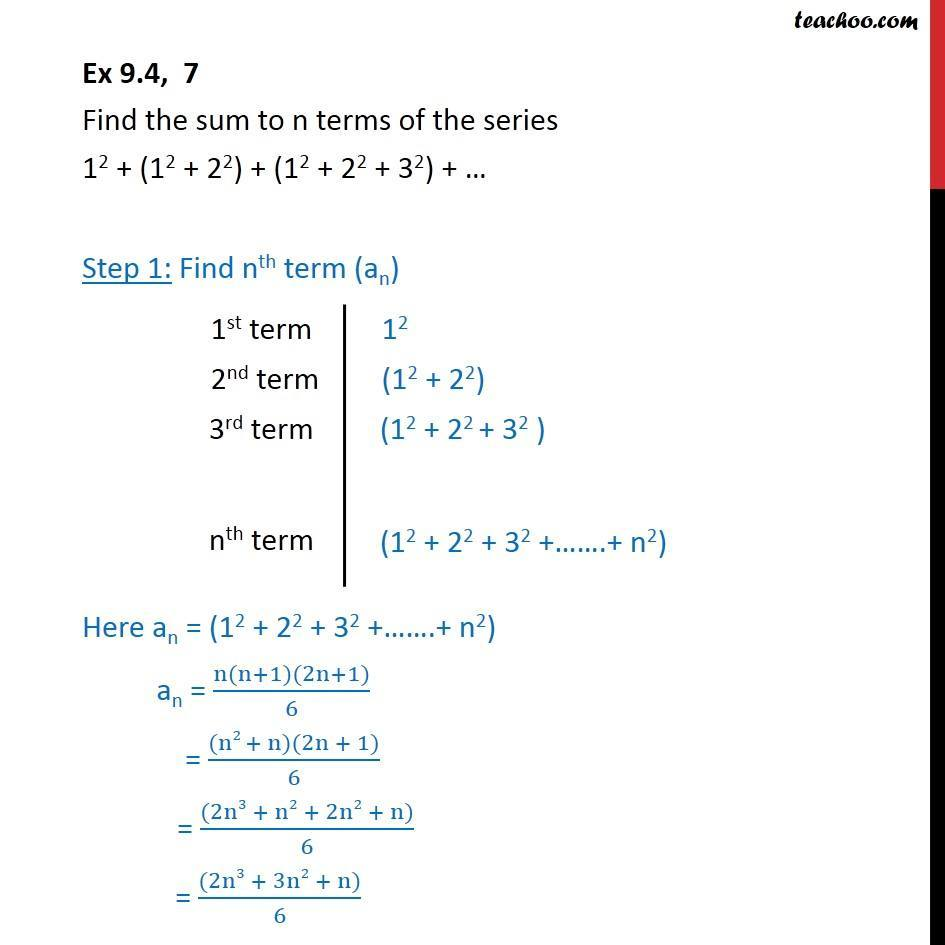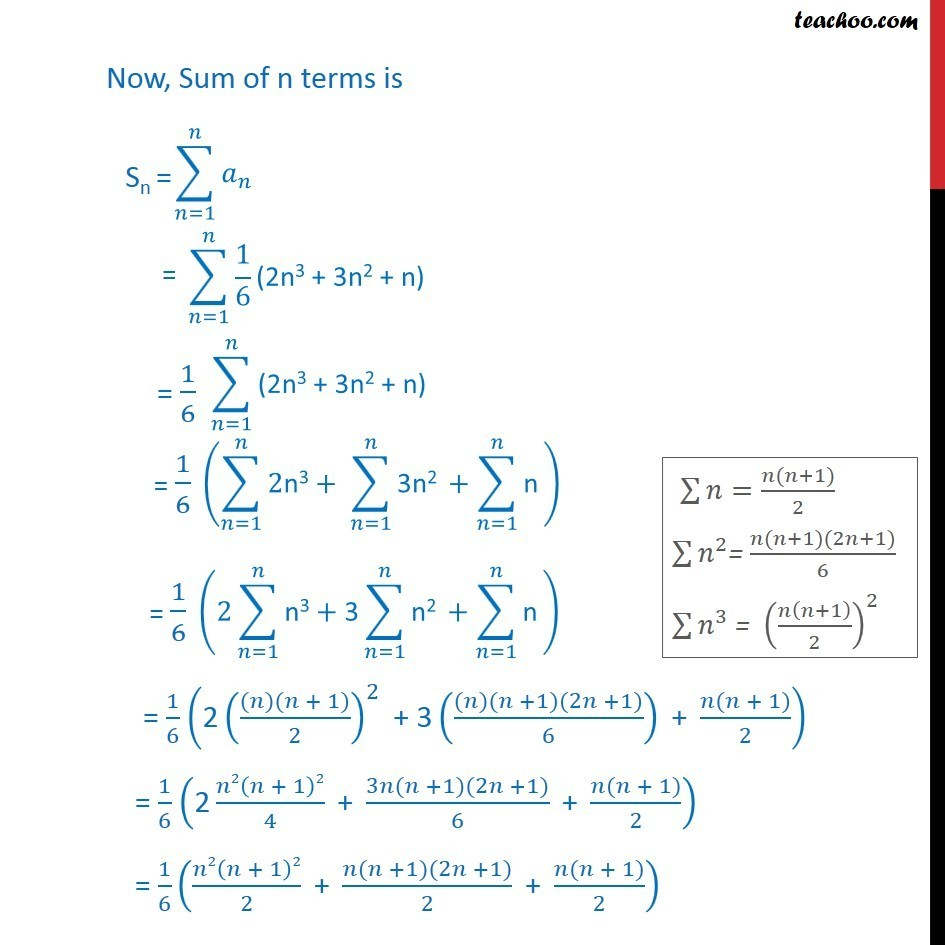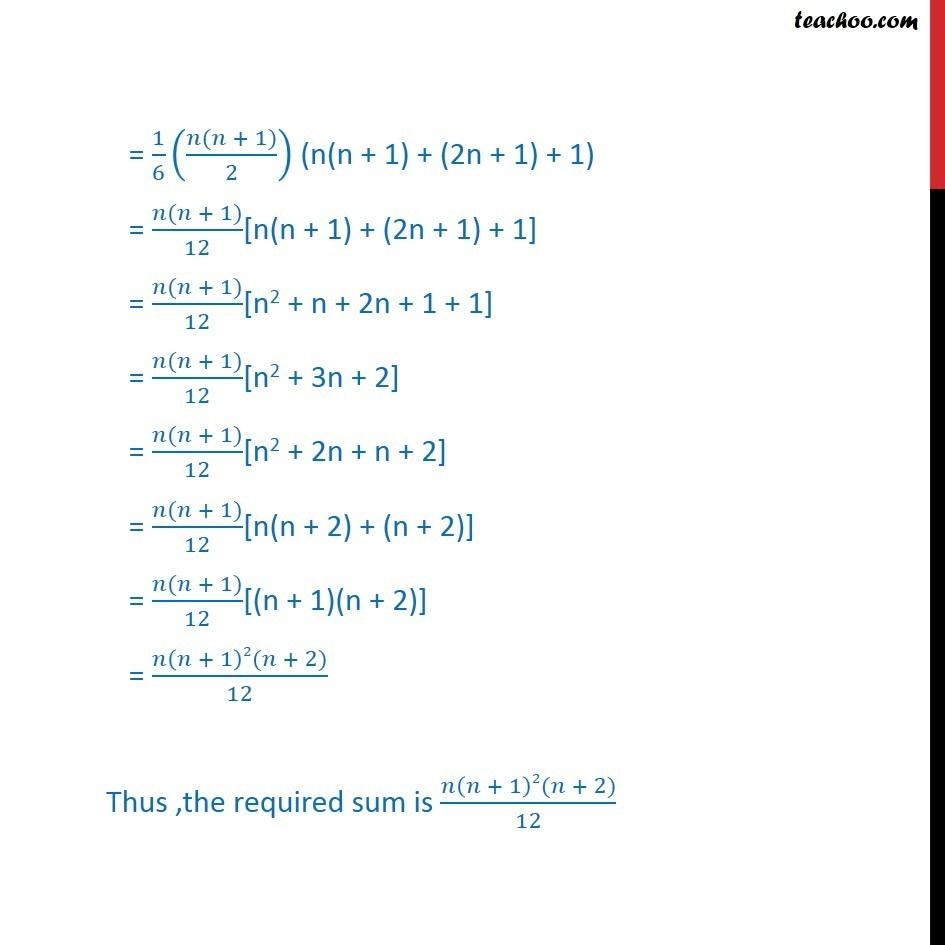Finding sum from nth number

Chapter 8 Class 11 Sequences and Series
Concept wiseLearn in your speed, with individual attention - Teachoo Maths 1-on-1 Class

### Transcript

Question 7 Find the sum to n terms of the series 12 + (12 + 22) + (12 + 22 + 32) + … Step 1: Find nth term (an) Here an = (12 + 22 + 32 +…….+ n2) an = (n(n+1)(2n+1))/6 = ((n2 + n)(2n + 1))/6 = ((2n3 + n2 + 2n2 + n))/6 = ((2n3 + 3n2 + n))/6 Now, Sum of n terms is = 1/6 ("2" (((𝑛)(𝑛 + 1))/2)^2 " + 3" (((𝑛)(𝑛 +1)(2𝑛 +1))/6)" + " (𝑛(𝑛 + 1))/2) = 1/6 ("2" 𝑛2(𝑛 + 1)2/4 " + " (3𝑛(𝑛 +1)(2𝑛 +1))/6 " + " (𝑛(𝑛 + 1))/2) = 1/6 (𝑛2(𝑛 + 1)2/2 " + " (𝑛(𝑛 +1)(2𝑛 +1))/2 " + " (𝑛(𝑛 + 1))/2) = 1/6 ((𝑛(𝑛 + 1))/2) (n(n + 1) + (2n + 1) + 1) = (𝑛(𝑛 + 1))/12[n(n + 1) + (2n + 1) + 1] = (𝑛(𝑛 + 1))/12[n2 + n + 2n + 1 + 1] = (𝑛(𝑛 + 1))/12[n2 + 3n + 2] = (𝑛(𝑛 + 1))/12[n2 + 2n + n + 2] = (𝑛(𝑛 + 1))/12[n(n + 2) + (n + 2)] = (𝑛(𝑛 + 1))/12[(n + 1)(n + 2)] = (𝑛(𝑛 + 1)2(𝑛 + 2))/12 Thus ,the required sum is (𝑛(𝑛 + 1)2(𝑛 + 2))/12